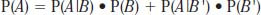NextPrevious

# What is the law of total probability?

The law of total probability can be written as follows: The probability that an event A will occur, P(A), is equal to the probability that event A and event B both occur, plus the probability that event A and event B' occur (or A occurs and B does not). Using the multiplication rule, this is written as:Close

This is a web preview of the "The Handy Math Answer Book" app. Many features only work on your mobile device. If you like what you see, we hope you will consider buying. Get the App